• 计算机专业书籍或者文章中，经常会用到代码，比如C语言代码，如何代码中添加注释，用图片的形式展示，这种排版很常见，但是用latex怎么实现？ 如图所示的效果，latex排版中如何实现？完整代码如下 \...
在计算机专业书籍或者文章中，经常会用到代码，比如C语言代码，如何在代码中添加注释，用图片的形式展示，这种排版很常见，但是用latex怎么实现？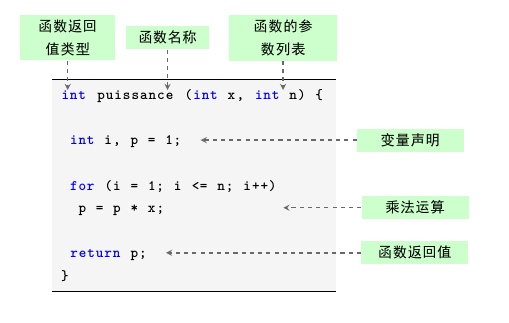如图所示的效果，在latex排版中如何实现？完整代码如下
\documentclass[svgnames]{book}

\usepackage{ctex}
\usepackage{tikz}
\usetikzlibrary{positioning}
\usepackage{listings}
\lstset{%
frame            = tb,    % draw frame at top and bottom of code block
tabsize          = 1,     % tab space width
numbers          = left,  % display line numbers on the left
framesep         = 3pt,   % expand outward
framerule        = 0.4pt, % expand outward
commentstyle     = \color{Green},      % comment color
keywordstyle     = \color{blue},       % keyword color
stringstyle      = \color{DarkRed},    % string color
backgroundcolor  = \color{WhiteSmoke}, % backgroundcolor color
showstringspaces = false,              % do not mark spaces in strings
}

\begin{document}

\begin{lstlisting}[language = C++, numbers = none, escapechar = !,
basicstyle = \ttfamily\bfseries, linewidth = .6\linewidth]
int!
\tikz[remember picture] \node [] (a) {};
!puissance!
\tikz[remember picture] \node [] (b) {};
!(int x,!
\tikz[remember picture] \node [] (c){};
!int n) {

int i, p = 1; !\tikz[remember picture] \node [] (d){};!

for (i = 1; i <= n; i++)
p = p * x; !\tikz[remember picture] \node [inner xsep = 40pt] (e){};!

return p; !
\tikz[remember picture] \node [] (f){};!
}
\end{lstlisting}
\begin{tikzpicture}[remember picture, overlay,
every edge/.append style = { ->, thick, >=stealth,DimGray, dashed, line width = 1pt },
every node/.append style = { align = center, minimum height = 10pt,	font = \bfseries, fill= green!20},
text width = 2.5cm ]

\node [above left = .75cm and -.75 cm of a,text width = 2.2cm]	(A) {函数返回值类型};
\node [right = 0.25cm of A, text width = 1.9cm]	(B) {函数名称};
\node [right = 0.5cm of B] (C) {函数的参数列表};
\node [right = 4.cm of d]  (D) {变量声明};
\node [right = 2.cm of e]  (E) {乘法运算};
\node [right = 5.cm of f]  (F) {函数返回值};

\draw (A.south) + (0, 0) coordinate(x1) edge (x1|-a.north);
\draw (B.south) + (0, 0) coordinate(x2) edge (x2|-b.north);
\draw (C.south) + (0, 0) coordinate(x3) edge (x3|-c.north);
\draw (D.west) edge (d.east) ;
\draw (E.west) edge (e.east) ;
\draw (F.west) edge (f.east) ;
\end{tikzpicture}

\end{document}
本案例中只是以一个简单的例子做演示，基本逻辑结构不会改变，在具体的实际应用中可以对样式进行调整，如果对画图还不是很了解的话，可以看看小编其他绘图相关的教程
展开全文latex 代码
• 该插件可让您深缩放图像绘制矢量注释。 该插件与图像成比例的SVG叠加层创建图纸。 用法 安装 您将同时需要OpenSeadragon和此插件。 您可以手动插件，也可以使用Bower或npm之类的软件包管理器进行安装。 npm...
• Unity 创建脚本时自动添加注释，内附详细教程，简单方便快捷
• 用Matplotlib进行可视化过程中，很多时候为了更直观地展现数据大小，会将具体的数值标注图形中，比如柱状图标明数值大小。这篇文章会以一个实际的案例，详细讲讲如何给数据注解，...在用Matplotlib进行可视化过程中，很多时候为了更直观地展现数据大小，会将具体的数值标注在图形中，比如在柱状图上标明数值大小。
这篇文章会以一个实际的案例，详细讲讲如何给数据加注解，同时也介绍一下一种比较骚的操作，即Matplotlib的内嵌图（把一张小图嵌入到一张大图中），学会这个后，你能够绘制出这种图形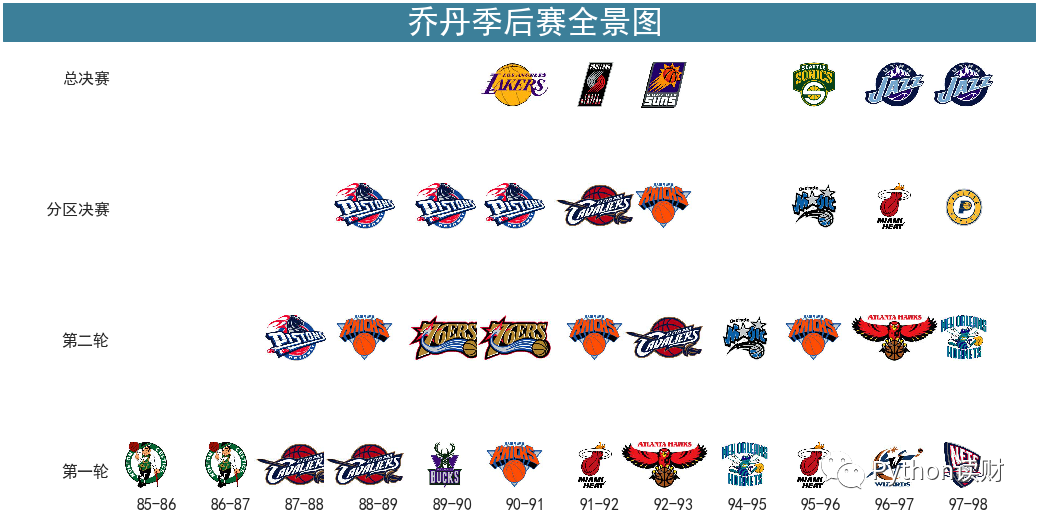数据注释
演示的数据集为2016年抵美（到达美国）人数排名前十的国家数据，包含国名和具体入境人数，人数的具体单位为百万人次
import pandas as pd
import numpy as np
import matplotlib.pyplot as plt
%matplotlib inline

plt.rcParams['font.sans-serif'] = 'SimHei'

x_data = ['加拿大','墨西哥','英国','日本','中国','德国','韩国','法国','巴西','印度']
y_data = [13.428347,12.255948,3.019111,2.352919,2.09833,1.322882,1.282097,1.127868,1.109066,0.874016]

有了数据后，可以非常快地画出一张柱状图
fig,ax = plt.subplots(figsize=(16,6))
ax.bar(x_data,y_data)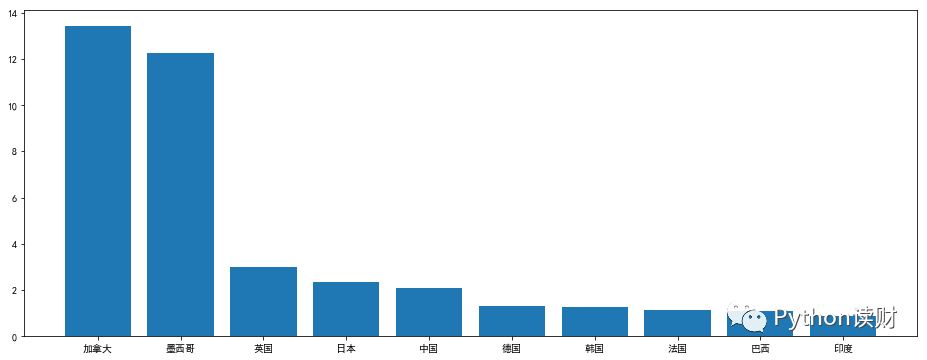接下来开始加注释，即在柱状图上显示具体数值。在Matplotlib中，为数据加上注释有两种方式，一种是使用ax.text()，另一种则是ax.annotate()。
ax.text()
ax.text()的主要作用是为图中加上一些text，也就是文字。不仅是能够加注释，只要指定了坐标，可以在图上的任何坐标加上text。
函数中的几个重要的参数，具体介绍一下
x：x的坐标y：y的坐标s：要加的文字rotation：文字旋转的角度fontsize：文字字体大小fontweight：文字字体粗细
需要注意的是，每次调用ax.text()只能生成一个Text对象，也就是说每次只能加一个注释，多个的话需要写循环生成。
所以一般加注释是这么一个流程：先确定注释的横纵坐标-->写循环调用ax.text()
fig,ax = plt.subplots(figsize=(16,6))
ax.bar(x_data,y_data)

# 循环生成text
# horizontalalignment参数设置注释居中显示
for x,y in zip(x_data,y_data):
ax.text(x,y+0.05,y,fontsize=14,horizontalalignment='center')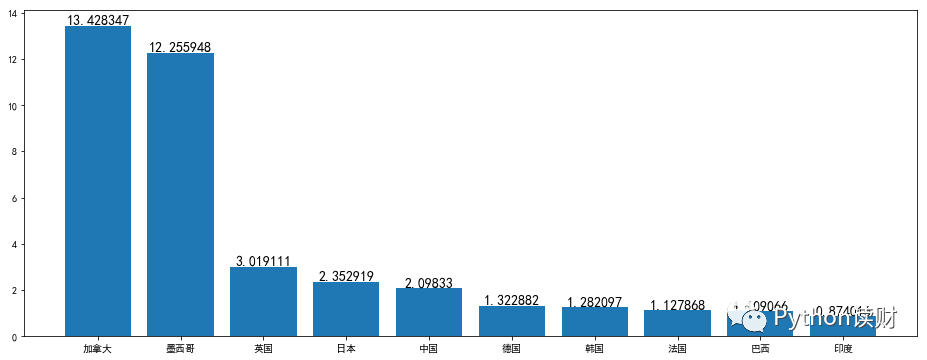关于图表美化方面，这里暂时不过多赘述。
ax.annotate()
相比于ax.text()，ax.annotate()更像是专门为做注释而生的，annotate便是注释的意思。
ax.annotate()的注释功能更强大，除了加入文本注释外，如果有需要还能够加上箭头→进行指示。
一些主要的参数如下：
s：注释文本xy：要加注释的数据点位置xytext：文本注释的位置，默认情况下为xyarrowprops：一个控制箭头的属性的dict，如果需要显示箭头，必须要设置
这里比较有意思的两个参数是xy和xytext，二者貌似没啥区别的样子，这个地方确实很容易产生困惑。
一般情况下，s和xy是必须要设置的参数，如果不指定，xytext默认和xy一致。
但如果要设置箭头的话，xy的坐标则定义了箭头的头部，xytext则指定箭头的尾部和文本注释的位置，实际画图来理解看看。
ax.annotate()和ax.text()的画图流程是一致的，都需要循环生成注释。
不设置箭头，简单加上注释
fig,ax = plt.subplots(figsize=(16,6))
ax.bar(x_data,y_data)

for x,y in zip(x_data,y_data):
ax.annotate(y,(x,y+0.05),fontsize=14,horizontalalignment='center')加上注释并设置箭头
fig,ax = plt.subplots(figsize=(16,6))
ax.bar(x_data,y_data)

# 箭头头部坐标(x,y)
# 箭头尾部坐标（注释坐标）为(x,y+1)
for x,y in zip(x_data,y_data):
ax.annotate(y,xy = (x,y),xytext = (x,y+1),fontsize=14,horizontalalignment='center',
arrowprops =dict(arrowstyle='->'))
# 将纵坐标范围扩大
ax.set_ylim([0,16])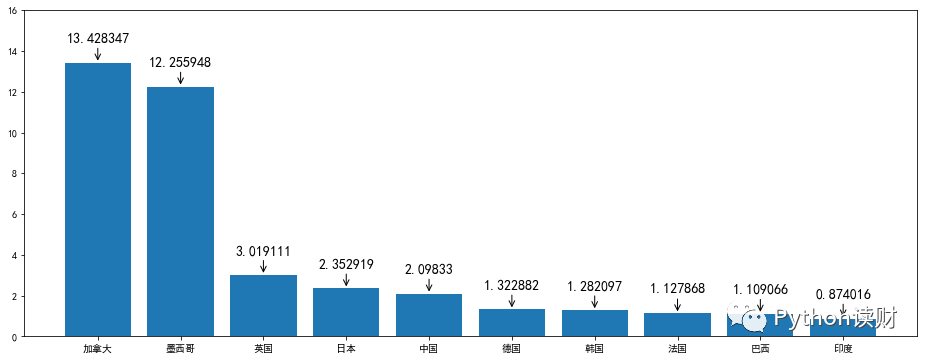设置了显示箭头之后，可以明显地看出，箭头是由xytext坐标指向xy的坐标的，所以，当你不需要设置箭头的时候，xytext设置的意义并不大。
内嵌图
内嵌图大家可能用的比较少，但这种图其实还是挺有用的。
拿上面的数据例子来说，柱状图显示了2016年抵美人数排名前十的国家的具体人数，如果这时想结合各大地域抵美人数的占比数据进行分析，该如何绘图？
一个比较直接的想法是用subplots子图来实现，比如上边显示柱状图，下边显示饼图，如下：
zhou_name = ['西欧','亚洲','南美洲','大洋洲','加勒比地区','中东地区','中美洲','东欧','非洲']
zhou_percent = [36.2,30.8,13.9,4.3,4.1,3.8,2.8,2.6,1.5]

fig,ax = plt.subplots(2,1,figsize=(16,12))
ax.bar(x_data,y_data)
for x,y in zip(x_data,y_data):
ax.annotate(y,(x,y+0.05),fontsize=14,horizontalalignment='center')

ax.pie(zhou_percent,labels=zhou_name,autopct='%1.1f%%')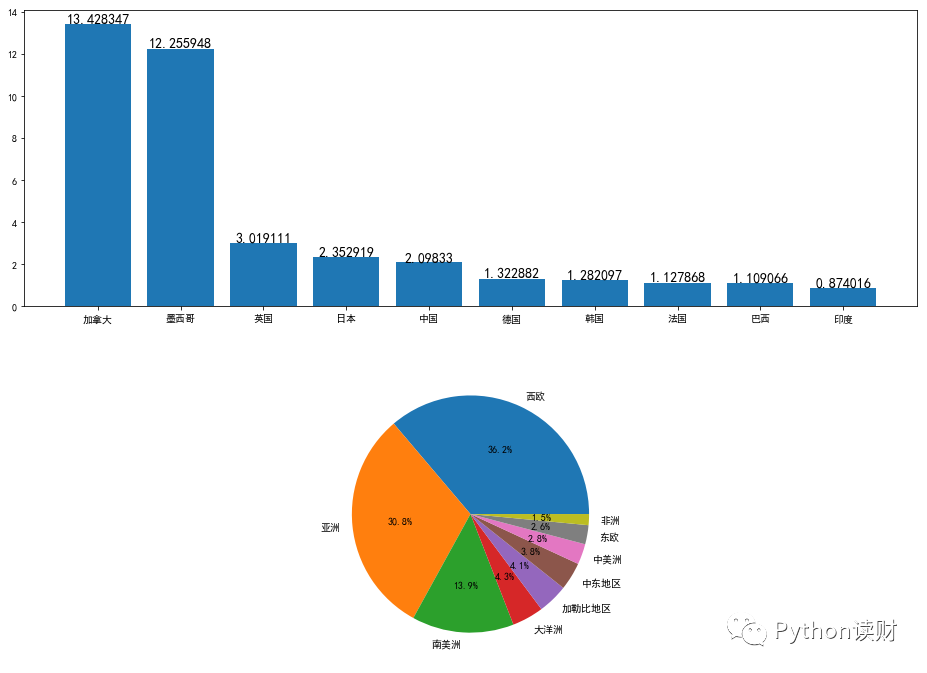这当然是可行的，但还有更好的方案，那便是内嵌图。注意到这里的柱状图右侧有很大的留白部分，如果把饼图放到柱状图右侧的留白部分会显得更加直观。
内嵌图有两种生成方式，一种是fig.add_axes()，另一种则是使用inset_axes()。
fig.add_axes()就是在原有的Figure上加上一个新的区域Axes，然后在这个区域中绘制图形。
使用这个方法的话需要指定新增的这个区域Axes在Figure中的相对位置和区域大小，输入参数均为相对于原来Figure的比例值，如下：
# left和bottom控制新Axes的位置
# width和height控制新Axes的大小（长宽）
# 这些均用相对数来表示，大小在0-1之间
left,bottom,width,height = [0.5,0.3,0.5,0.5]

fig,ax1 = plt.subplots(figsize=(16,6))
ax1.bar(x_data,y_data)
for x,y in zip(x_data,y_data):
ax1.annotate(y,(x,y+0.05),fontsize=14,horizontalalignment='center')

ax2.pie(zhou_percent,labels=zhou_name,autopct='%1.1f%%')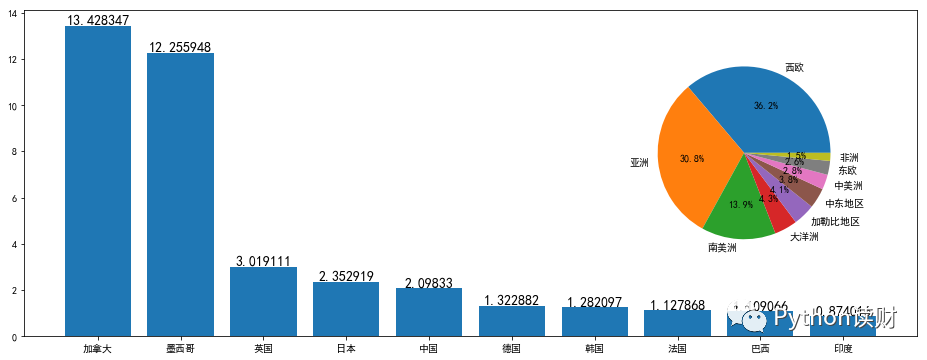inset_axes
相比于fig.add_axes()需要对相对位置进行调试，使用inset_axes()进行绘图则可以方便进行定位。
inset_axes中的位置由参数loc设置，可以用字符串控制，也可以输入数字，具体如下：

'upper right'  : 1
'upper left'   : 2
'lower left'   : 3
......
'upper center' : 9
'center'       : 10

# 使用前需要先导包
from mpl_toolkits.axes_grid1.inset_locator import inset_axes

fig,ax1 = plt.subplots(figsize=(16,6))
ax1.bar(x_data,y_data)
for x,y in zip(x_data,y_data):
ax1.annotate(y,(x,y+0.05),fontsize=14,horizontalalignment='center')

# 将内嵌图置于右侧，宽度和长度分别为相对长度
ax2 = inset_axes(ax1,width = '60%',height = '60%',loc='right')
ax2.pie(zhou_percent,labels=zhou_name,autopct='%1.1f%%')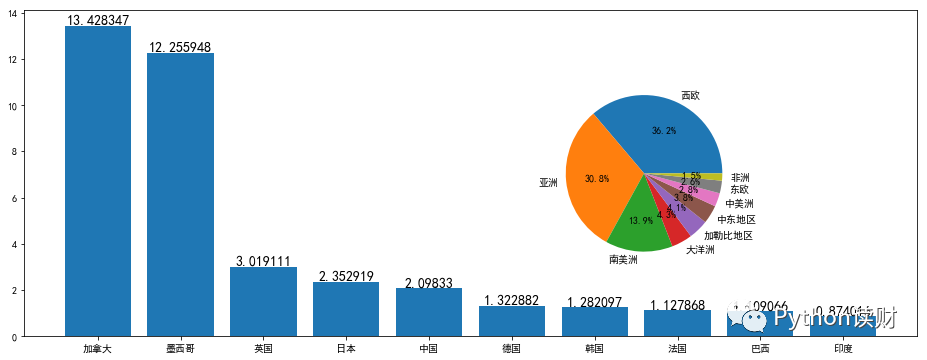美化方面这里就不再多讲了，具体可以参照这篇文章如何用 Matplotlib 画一张好看的图。推荐阅读：
一文读懂高并发情况下的常见缓存问题
用 Django 开发基于以太坊智能合约的 DApp
一文读懂 Python 分布式任务队列 celery
5 分钟解读 Python 中的链式调用
用 Python 创建一个比特币价格预警应用▼ 点击即享阿里云产品0.9折优惠起

展开全文• import numpy as np import matplotlib.pyplot as plt fig, ax = plt.subplots() x = np.arange(0.0, 5.0, 0.01) y = np.cos(2*np.pi*x) line, = ax.plot(x,y,lw=2) ax.annotate('local max', # ⽂本内容 ...
import numpy as np
import matplotlib.pyplot as plt

fig, ax = plt.subplots()
x = np.arange(0.0, 5.0, 0.01)
y = np.cos(2*np.pi*x)
line, = ax.plot(x,y,lw=2)

ax.annotate('local max', # ⽂本内容
xy=(2, 1), # 箭头指向位置
xytext=(3, 1.5), # ⽂本位置
arrowprops=dict(facecolor='black', shrink=0.05)) # 箭头

ax.annotate('local min',
xy = (2.5,-1),
xytext = (4,-1.8),
arrowprops = dict(facecolor = 'black',
width = 2, # 箭头宽度
shrink = 0.1)) # 箭头两端收缩的百分⽐（占总⻓）

ax.annotate('median',
xy = (2.25,0),
xytext = (0.5,-1.8),
arrowprops = dict(arrowstyle = '-|>'), # 箭头样式
fontsize = 20)

ax.set_ylim(-2, 2)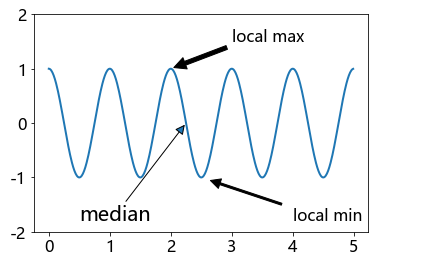展开全文python 数据可视化 matplotlib
• 一步为Jupyter Notebook添加图片注释 1、对要添加图片截图。 2、选择Markdown，直接复制后，点击运行。 3、图片就可以显示出来了。
一步为Jupyter Notebook添加图片注释
1、对要添加的图片截图。 2、选择Markdown，直接复制后，点击运行。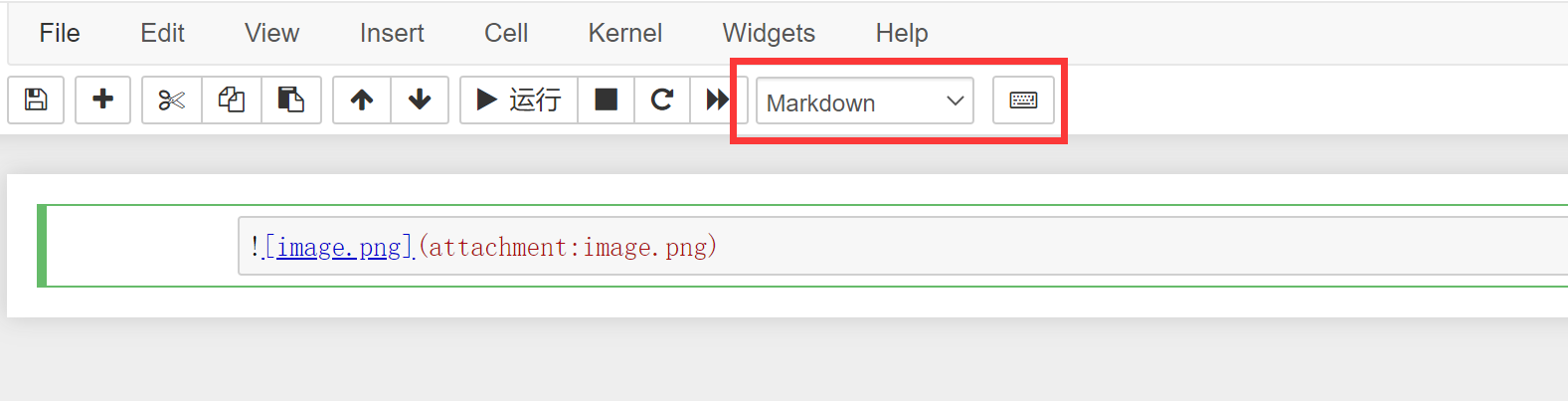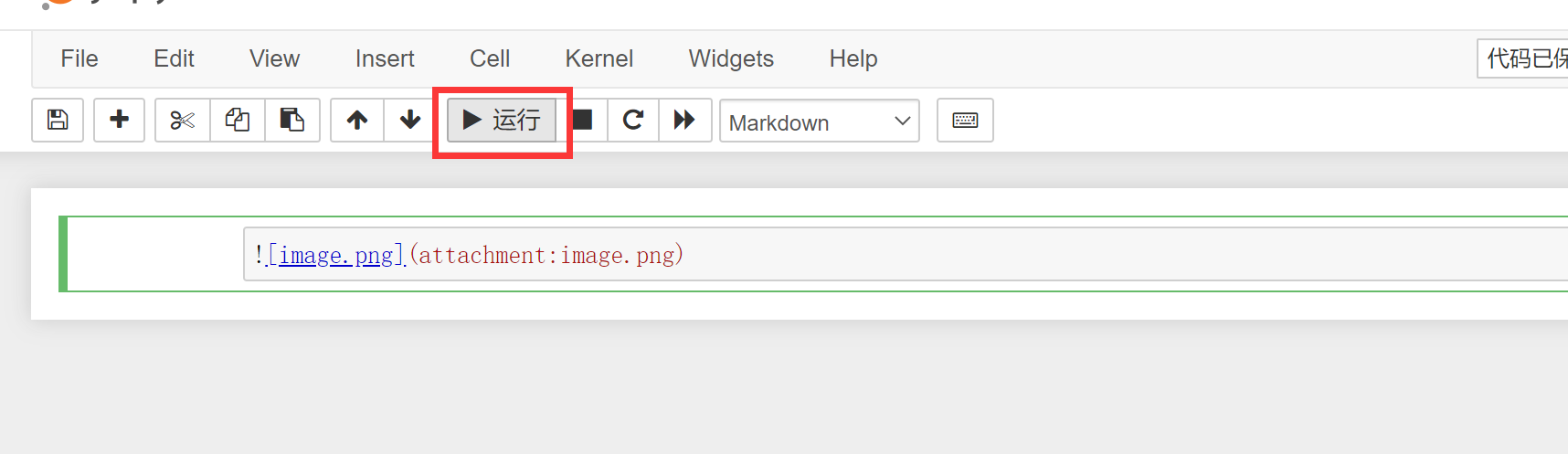3、图片就可以显示出来了。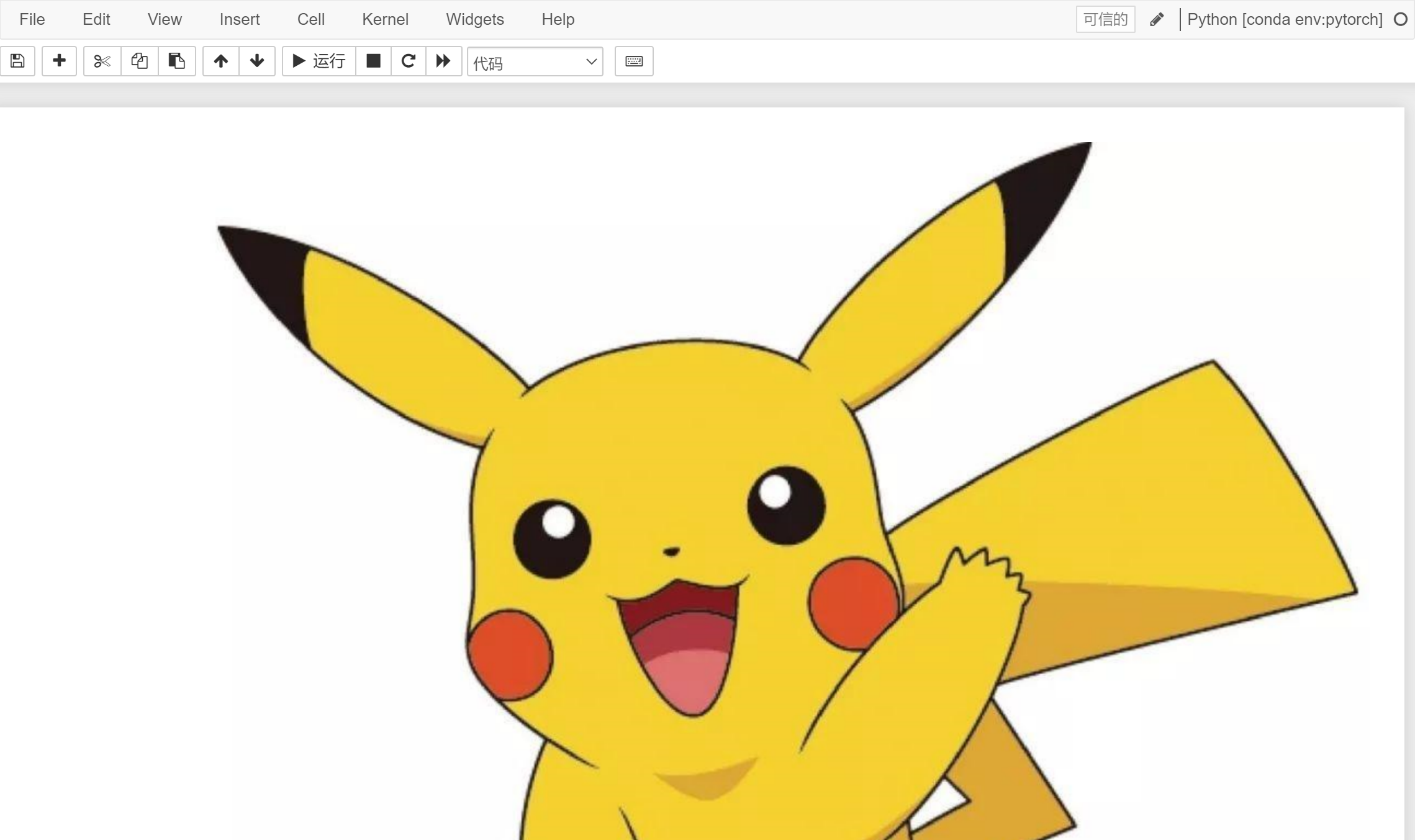展开全文markdown python
• 比较精细的C#版画图+注释功能，画图和注释都是一个层，中间可以任意拖拉修改
• Mozilla的PDFJS之的FabricJS层上添加注释 产品特点 支持多页PDF 免费绘画工具 添加文字 添加箭头 添加矩形 添加图片 改变颜色 更改画笔大小 更改字体大小 每个对象都可以调整大小 将所有画布数据序列化为JSON并...
• 详情见链接： https://ww2.mathworks.cn/help/matlab/ref/annotation.htmlmatlab
• excel图坐标轴添加注释图片添加到Excel注释 (Add a Picture to an Excel Comment)Things have been hectic this week. I got a new laptop, with Window 8, and have spent hours installing software, and ...java python linux 编程语言 excel
• 双击左键，写入注释行即可，还可以箭头指向对象； 下面写入“hello” ;labview
• 对于刚换IDEA开发的开发者来说，都逃不开如何设置类注释和方法注释的快捷键。 IDEA类注释有两种方法，第一种是创建类...方法注释与第二种类注释本质是一样的操作。 此文将详细介绍这两种配置，并附上相当详细的截图java idea
• html5，js编写，对图片进行热点标注，可以任意区域进行标注，添加注释，最后可以一键保存成图片！！！
• Revit的注释选项卡中包含6个面板，分别为：尺寸标注、详图、文字、标记、颜色填充、符号。一、文字标注文字标注的内容一般是手动输入的文字，与项目文件或模型图元的属性没有直接关联，...生成文字后可以功能区中...
• 1.idea类注释 打开：file-&gt;setting-&gt;Editor-&gt;Filr and Code Templates-&gt;Includes-&gt;File Header    类注释模板： /** * @Description: TODO * @author ${USER} * @date ...idea 模板 • 1、Template text内容 * * @author$User$* @description$description$* @date$datetimeparam$* @return **/ 图片如下： 2、param单行显示脚本 groovyScript("def result=''; def params=\"${_1}\"....java
• 而且大家用起来一般还只停留阅读的使用，要是涉及到编辑、修改就会束手无策了，其实伴随着使用和下载，大家已经是对PDF格式有了不少了解，想要编辑、修改也是件简单的事情，那么PDF文件注释要怎么？...
• 一份简单的latex中文论文教程，想看效果的话可以先看一下我的教程 https://blog.csdn.net/tstarysy/article/details/79688289latex
• 关于Latex的安装，这里我用到了Texstudio，大家戳下面的链接按照步骤进行下载：CSDN：最简单傻瓜式的 Latex+Texstudio 安装教学...Texstudio/winEdit里或者直接 http://overleaf.com网页版里进行...
• （ios开发）MapKit地图上添加注释 1. 添加到map view的子视图不会随地图的移动而移动，map view会固定其子视图的位置。如果要添加随着地图移动的子视图，可以使用annotations和overlays。a地图
• annotator, web的注释工具 选择文本图像或者( 近)，然后添加注释 注释注释器是用于浏览器中构建注释应用程序的JavaScript库。 它提供了一套可以互操作的工具，用于网页中标注内容。 有关简单演示，请访问 ...
• 假设，我们想要看不同日期，收益和成本的变化，该怎么绘制图片。我们只需要两次plot（）绘制出折线图。 from matplotlib import pyplot as plt plt.rcParams['font.sans-serif'] = ['SimHei'] # 用来正常显示中文...
• 公众号后台回复“图书“，了解更多号主新书内容作者：易执来源：Python读财用Matplotlib进行可视化过程中，很多时候为了更直观地展现数据大小，会将具体的数值标注图形中，比如...数据可视化 css python 数据分析 js
• 首先展示一下 我添加注释的结果， 　 下面是我的做法： 　 　Adobe Acrobat 中打开Sample.pdf文档，点开文档右边的“工具”-“内容”-选择“编辑对象”，鼠标光标变成实心箭头+右下角小方框。 　文档空白...
• 1 xml文件 xml是Extensible Markup Language即可扩展标记...”，即可将注释写入到“注释内容”的位置，如图1所示。 图1 手动添加注释 3 自动添加注释 可以通过菜单栏或者快捷按键自动添加注释 3.1 菜单栏添.XML文件
• 一，效果图：  二，代码： \begin{figure}[t] \centering  \begin{tabular}{cc} \begin{minipage}[t]{2in} \includegraphics[width=1.8in]{gestalt_theory/similarity.jpg} \caption{Similarit...
• 编写代码时，我们经常添加文档注释，来记录代码的作用。 本文介绍三种常用的文档注释。 文档注释 创建文件的时自动添加注释内容，声明该文件的作用和创建时间。 打开设置 找到相应设置 代码内容中输入 /**...java idea
• 和python基础配合着看 https://editor.csdn.net/md/?articleId=110882085python
• 博客http://blog.csdn.net/qq_34258009/article/details/79581756，代码添加注释
• .wxml文件中加入注释的方法： ctrl+/ :快速添加和...例：先输入所的文字，选中注释文字，按 ctrl+/ 键 <!--pages/welcome/welcome.wxml--> <!-- class给每个需要样式的组件加入样式名字 --> ...css js...